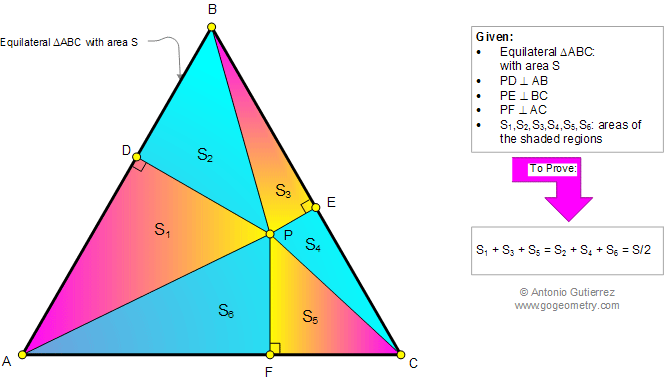###### Online Geometry Problem 876: Equilateral Triangle, any Point, Perpendicular, Right Triangle Area, Sum of Areas. Level: High School, College, Mathematics Education
 The figure below shows an equilateral triangle ABC of area S. P is any point and PD, PE, and PF are perpendicular to AB, BC, and AC, respectively. If S1, S2, S3, S4, S5, and S6 are the areas of the shaded regions, prove that S1+S3+S5 = S2+S4+S6 = S/2. This entry contributed by Ajit Athle.Home | Search | Geometry | Problems | All Problems | Open Problems | Visual Index | 10 Problems | 871-880 | Triangle | Equilateral Triangle | Perpendicular | Right Triangle | Area | Triangle area | Email | Solution / comment | By Antonio Gutierrez